# 3D FEM simulation of Electromagnetic actuator with Ferrofluid

HOME / Applications / 3D FEM simulation of Electromagnetic actuator with Ferrofluid

### Applications

Used Tools:## Introduction

Linear electromagnetic actuators with solenoid type, are widely used in industrial applications. They are part of various electromagnetic devices based on different controlling mechanisms; they are used in electromagnetic valve actuation systems, fuel injection, exhaust gas recirculation systems, washing machines, etc.

In order to develop an electromagnetic actuator with optimal actuation force, high reliability and low energy consumption, a new generation of actuators design has been developed with the addition of a ferrofluid in the working gap. Such type of fluid with an increased magnetic permeability could significantly reduce the magnetic reluctance of the actuator.

Ferrofluids, also known as magnetic fluids, are a special category of smart Nano-materials. The fluid exhibits both fluidity and superparamagnetism, with strong magnetic dipoles which can be effectively controlled by magnetic fields . Nowadays, ferrofluid liquids are exploited in numerous industrial and laboratory applications. They are usually formed of a colloidal solution with very fine ferromagnetic particles diluted in sufficiently viscous carrier liquids. Higher viscosity of these liquids ensures their relatively good spatial and temporal homogeneity. Some of their physical parameters (permeability and viscosity) strongly depend on external magnetic field.

Electromagnetic systems with ferrofluid nanoscale materials are characterized by a better work performance and a reduced energy consumption. One of their prospective technical applications is represented by classical electromagnetic actuators where the working-space is filled with ferrofluidic liquid, which can offer more interesting magnetic force enhancements. The total magnetic resistance of the actuators is lower, and the required magnetic flux density may be achieved with lower field current.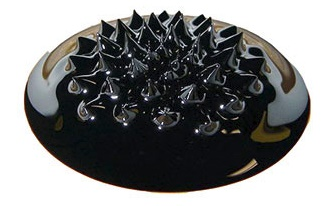Figure 1 - Ferrofluid 

## Problem description

The studied electromagnetic actuator consists of a stationary ferromagnetic core surrounding a copper DC coil, a movable ferromagnetic plunger and a working gap filled with ferrofluid (as shown in Figure 2).  The coil consists of 500 turns copper wire with a diameter 1mm. The material of core and plunger is Carbon Steel 12040 with B-H characteristic.

The working principle of the actuator is based on exciting the ferromagnetic circuit with a coil carrying DC current, which will produce a magnetic field (B) that pulls the plunger through magnetic force (Fm). If this force is higher than the sum of various resistances (friction, hydrodynamic resistance, external force), the plunger then moves.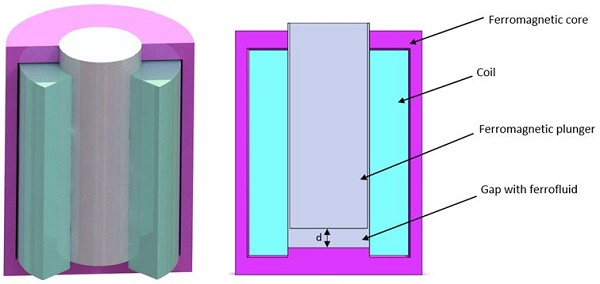Figure 2 - Cross sectional view of the studied design

Table 1 - Component` Dimensions

 Part Dimensions (mm) Core Diameter: 48 Height: 63 Thickness: 3 Coil Diameter: 41 Height: 53 Thickness: 10 Plunger Diameter: 20 Height: 53

## Material properties

Table 2 - Material properties

 Density (Kg/) Magnetic permeability Electrical conductivity (S/m) Copper (Cu) 8900 0.99 6 E+07
During this study, the operational static characteristics are determined for actuator with ferrofluid (µ >1) and without ferrofluid (µ=1) in the working gap. Two models will be discussed: the first model with Linear Carbon Steel 12040 material (Core and plunger) with magnetic permeability µ=1000 and the second model with nonlinear material characteristic defined by the next BH curve: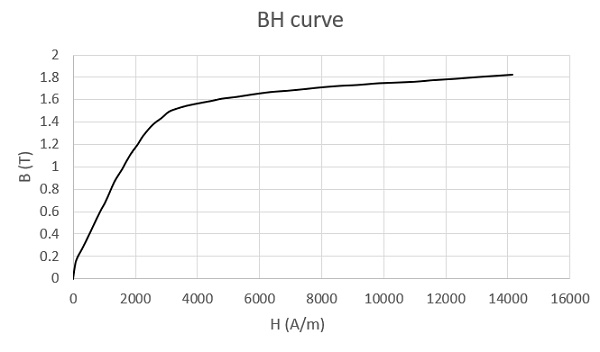Figure 3 - BH curve of Carbon steel 12040

## Meshing

The meshed model is shown in Figure 4. Fine mesh control was applied to whole model for accurate results.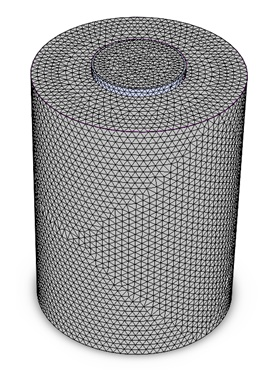Figure 4 - Meshed model

To evaluate the effect of the Ferrofluid on the behavior of actuator for different plunge positions, a succession of simulations was executed for a range of the Ferrofluid permeability between 1 and 50. The obtained results are shown below.

## Magnetic field results

### Case 1: Linear ferromagnetic Carbon steel

For the case of linear ferromagnetic Carbon Steel material, the flux density and magnetic field distribution for different values of Ferrofluid permeability and plunger positions are shown by the cross-sectional view plots in the figure 5,6 and 7.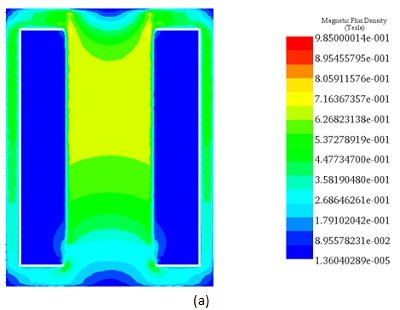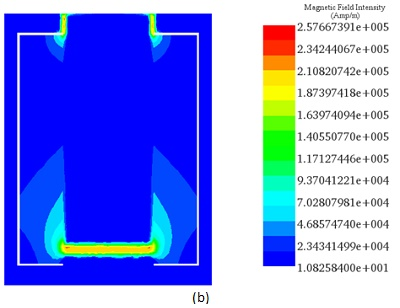Figure 5 - a) Magnetic flux density and b) Magnetic field distribution for µ=1, d=2.5 mm.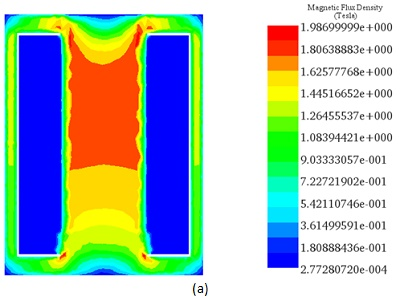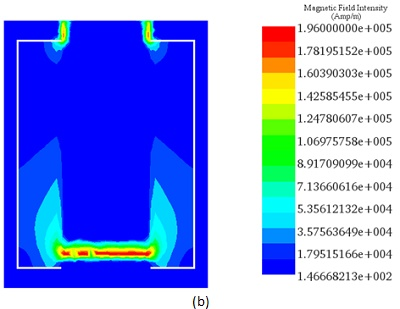Figure 6 - a) Magnetic flux density and b) Magnetic field distribution for µ=5, d=2.5 mm.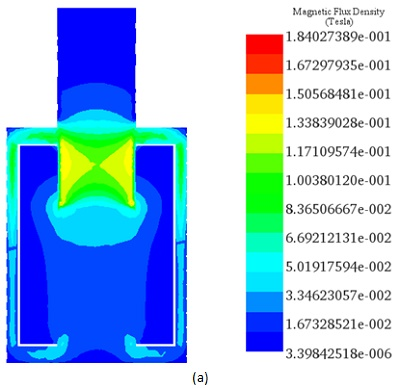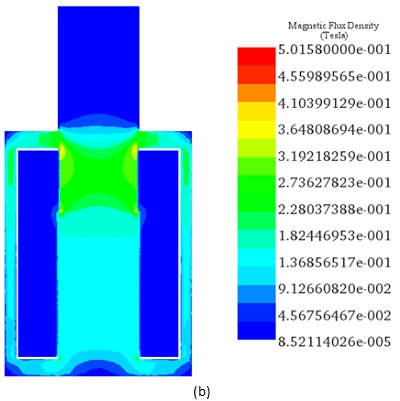Figure 7 - a) Magnetic flux density for µ=1 and b) µ=5 at d=35 mm.

Table 3 - Comparative table of Flux density results between EMS and Ref .

 Magnetic flux results EMS Reference  µ=1, d=2.5 mm 0.985 0.985 µ=5, d=2.5 mm 1.98 1.71 µ=1, d=35 mm 0.184 0.184 µ=5, d=35 mm 0.5 0.499

### Case 2: Non-Linear ferromagnetic Carbon steel

For the case of Non-linear ferromagnetic Carbon Steel material, the simulations revealed the magnetic flux results below: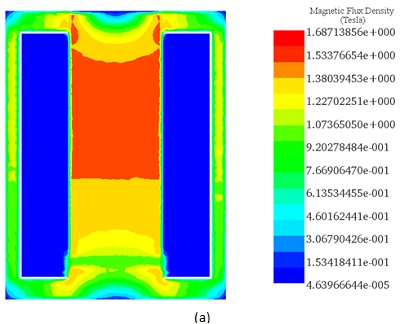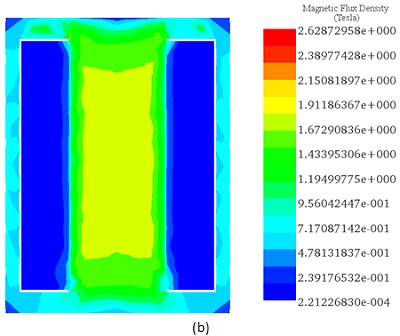Figure 8 - a) Magnetic flux density for µ=5 and b) µ=50 at d=2.5 mm.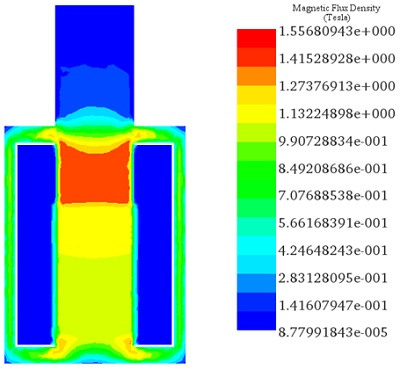Figure 9 - Magnetic flux density for µ=50 at d=35 mm.

## Magnetic force results

### Case 1: Linear ferromagnetic Carbon steel

For the case of linear problem, the generated electromagnetic force is evaluated according to relative magnetic permeability of ferrofluid at different working gaps.

The variation of force versus the plunger position, for a ferrofluid permeability of 50, is shown by the Figure 10. The variation of plunger position acts inversely on the generated magnetic force. This last achieves considerable values for lower ferrofluid gaps.

For a fixed plunger position set to d=2.5 mm from the core base, the obtained force results are presented by the figure 11. It is noticed that with higher magnetic permeabilities of the ferrofluid, the generated force achieved higher values. The maximum value of electromagnetic force is Fmax73 N with µ = 50.A comparison between EMS and Reference  results show a good coincidence between them.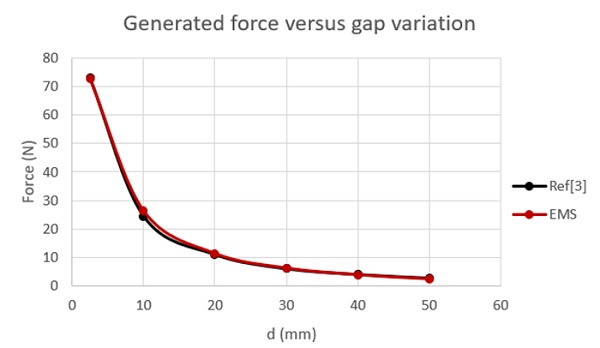Figure 10 - EMS and Reference  results for different plunger positions for µ=50.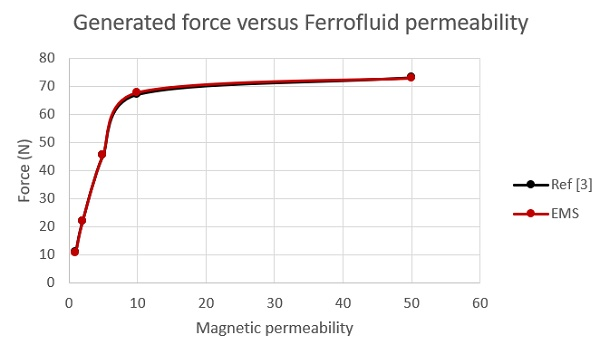Figure 11 - EMS and Reference  results for different ferrofluid permeabilities at plunger position of d=2.5 mm.

### Case 2: Non-Linear ferromagnetic Carbon steel

For the case of Non-linear ferromagnetic carbon steel, a succession of simulations was executed for different plunger positions and Ferrofluid permeability values, to compute the generated force under the effect of the Ferrofluid gap.

The variation of force versus the plunger position, for a ferrofluid permeability of 5, is shown by the Figure 12. The variation of plunger position acts inversely on the generated magnetic force. This last achieves negligible values at large ferromagnetic gaps.

For a fixed plunger position set to d=2.5 mm from the core base, the obtained force results are presented by the figure 13. In small gaps electromagnetic force is increasing significantly at range of relative magnetic permeability from 1 to 5. With higher relative magnetic permeability, electromagnetic force is decreasing. The maximum value of electromagnetic force is Fmax29 N with µ = 5.A comparison between EMS and Reference  results show a good coincidence between them.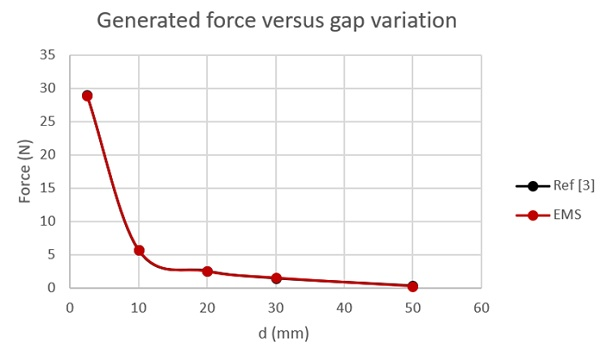Figure 12 - EMS and Reference  results for different plunger positions for µ=5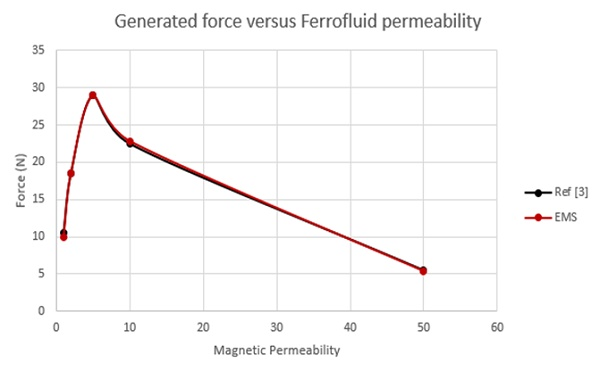Figure 13 - EMS and Reference  results for different ferrofluid permeabilities at plunger position of d=2.5 mm.

## Conclusion

The use of Ferrofluid materials with increased magnetic permeability could significantly decreases the magnetic reluctance of the systems. Ferrofluid is characterized with high magnetization saturation with no remanence. Ferrofluid in the working gap of electromagnetic actuators increases electromagnetic force and thus, with such setting, greater force could be obtained with more compact actuator.

Ferrofluid perfectly adapts to any geometry and could moves through very small channels. It is used to eliminate or substantially reduce the noise caused by the actuator plunger striking the core. Furthermore, magnetic flux leakage is usually a significant problem associated with solenoid actuators. This leakage can be reduced through the use of the ferrofluid in magnetic circuit of the actuator.